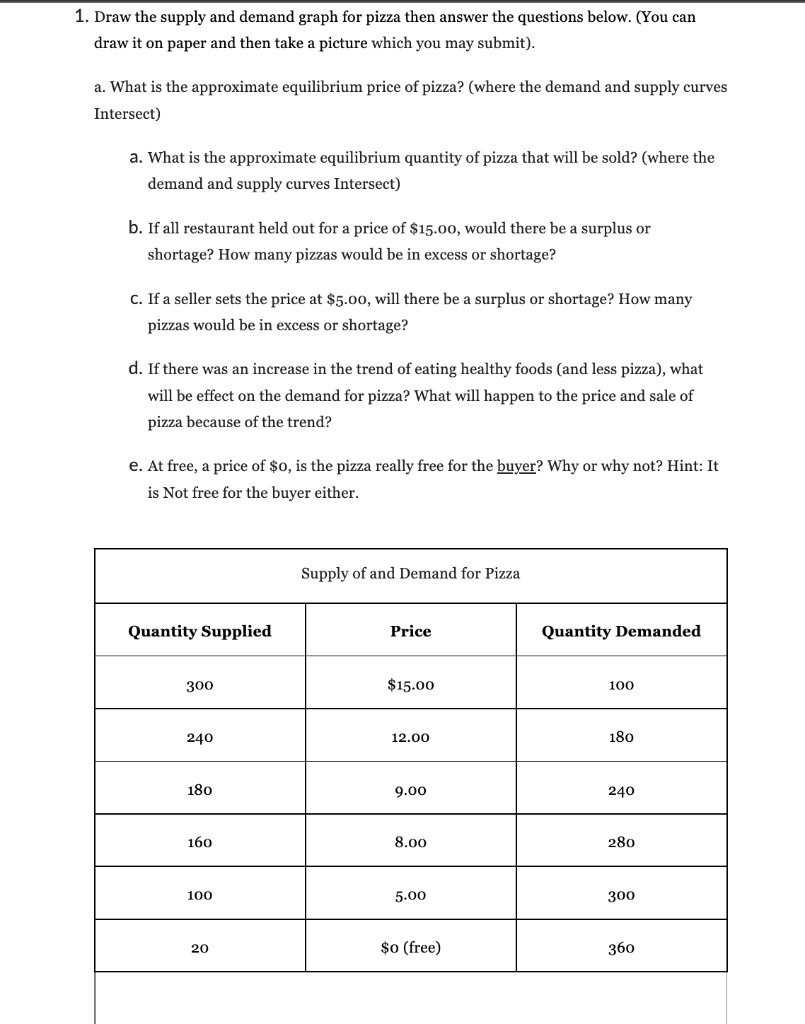# (Solved): 1. Draw the supply and demand graph for pizza then answer the questions below. (You can draw it on ...1. Draw the supply and demand graph for pizza then answer the questions below. (You can draw it on paper and then take a picture which you may submit). a. What is the approximate equilibrium price of pizza? (where the demand and supply curves Intersect) a. What is the approximate equilibrium quantity of pizza that will be sold? (where the demand and supply curves Intersect) b. If all restaurant held out for a price of \( \\$ 15.00 \), would there be a surplus or shortage? How many pizzas would be in excess or shortage? c. If a seller sets the price at \( \\$ 5.00 \), will there be a surplus or shortage? How many pizzas would be in excess or shortage? d. If there was an increase in the trend of eating healthy foods (and less pizza), what will be effect on the demand for pizza? What will happen to the price and sale of pizza because of the trend? e. At free, a price of \( \\$ 0 \), is the pizza really free for the buyer? Why or why not? Hint: It is Not free for the buyer either.

We have an Answer from Expert